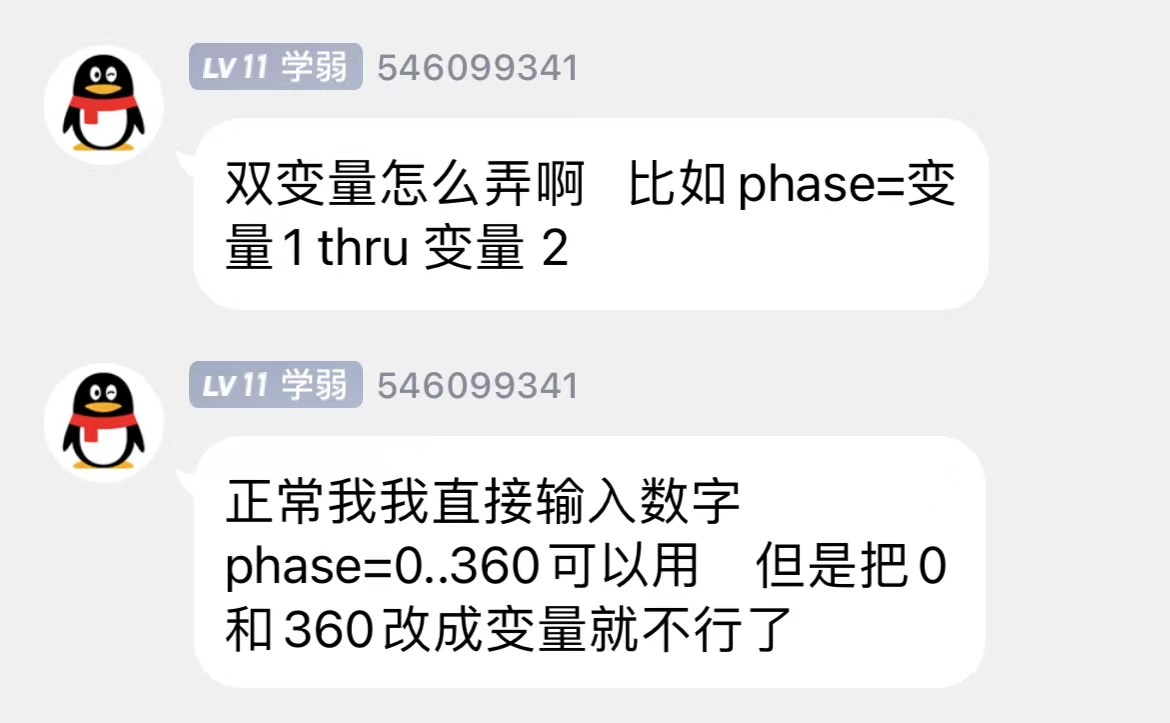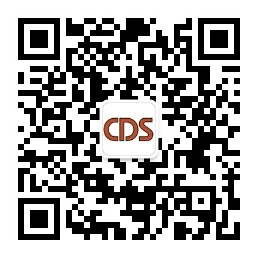# MA如何在文本中插入变量的值？phase = 0..360phase = “0..360” 等价，也就说等于号后面是字符串，如果直接改为 phase = “\$A..\$B”，则报错，因为双引号是定义字符串，双引号里面所有东西系统都视作字符串，因此是不能正确识别变量的。

`Setvar \$A = ("Input the Phase from")     //输入第一位相位`
` Setvar \$B = ("Input the Phase to")       //输入第二位相位 `
`Addvar \$A = ".."                         //在原变量A后面增加字符串.. `
`AddVar \$A = \$B                           //再次在变量A后面增加字符串变量B `
`Assign Effect 1 /Phase = \$A              //为效果1修改相位值`

`SetVar \$sequen = "on executor "                //定义字符串变量sequen，注意字符串最后有一个空格 `
`AddVar \$sequen = ("Input the sequence number") //在变量sequen后面增加输入的数值 `
`Select @                                       //选择执行器 `
`Assign Cue 1 /cmd = \$"sequen"                  //修改cue 1的CMD，注意字符串变量双引号的位置`